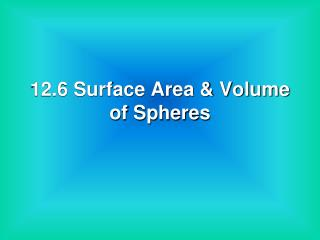DownloadDownload Presentation12.6 Surface Area & Volume of Spheres

# 12.6 Surface Area & Volume of Spheres

Télécharger la présentation## 12.6 Surface Area & Volume of Spheres

- - - - - - - - - - - - - - - - - - - - - - - - - - - E N D - - - - - - - - - - - - - - - - - - - - - - - - - - -
##### Presentation Transcript

1. 12.6 Surface Area & Volume of Spheres

2. Definitions • Sphere – the locus of points in space that are a given distance from a given point. (looks like a ball) • Center of a Sphere – the given point in the middle. • Radius of a Sphere – segment from the center to a point on the sphere. • Chord of a Sphere – a segment whose endpoints are on the sphere. • Diameter of a Sphere – a chord that goes through the center.

3. Parts of a Sphere C is the center of the sphere. AB is a diameter. CB & AC are radii. DE & AB are chords. C A B E D

4. Thm 12.11 – Surface Area of a Sphere S = 4r2 (it takes 4 circles to cover a sphere) Why isn’t there a lateral area formula? Because spheres have no bases! Ex: Find the surface area of a sphere with a diameter of 8 cm. S = 4(4)2 S = 4(16) S = 64 cm2

5. More Definitions • Great Circle of a Sphere – the cross section of a sphere sliced by a plane through its center. • Hemisphere – ½ of a sphere. ** Every great circle splits a sphere into 2 hemispheres.

6. C = 2r 16 = 2r 16 = 2r 8 m = r S = 4r2 S = 4(8)2 S = 4(64) S = 256 m2 Ex: The circumference of a great circle of a sphere is 16 m. What is the surface area of the sphere?

7. Thm 12.12 – Volume of a Sphere Ex: Find the volume of a sphere with a radius of 3 ft. V = 36 ft3